# Array Sorting Searching Sorting Algorithms A sorting algorithm

• Slides: 27Array, Sorting, Searching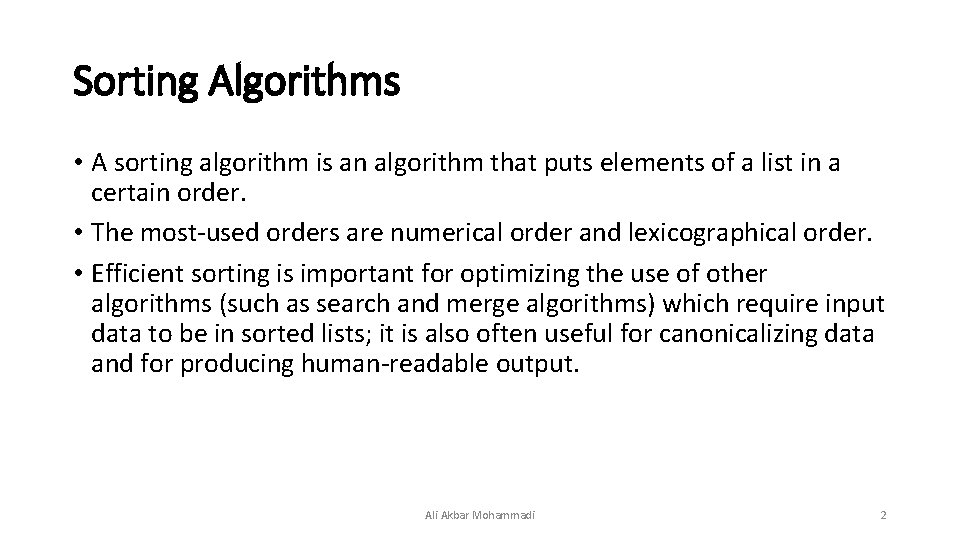Sorting Algorithms • A sorting algorithm is an algorithm that puts elements of a list in a certain order. • The most-used orders are numerical order and lexicographical order. • Efficient sorting is important for optimizing the use of other algorithms (such as search and merge algorithms) which require input data to be in sorted lists; it is also often useful for canonicalizing data and for producing human-readable output. Ali Akbar Mohammadi 2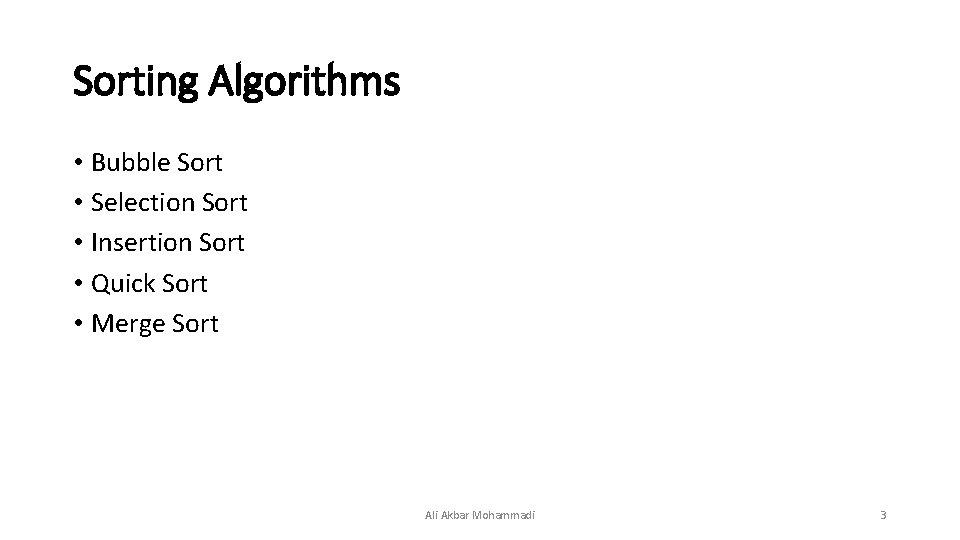Sorting Algorithms • Bubble Sort • Selection Sort • Insertion Sort • Quick Sort • Merge Sort Ali Akbar Mohammadi 3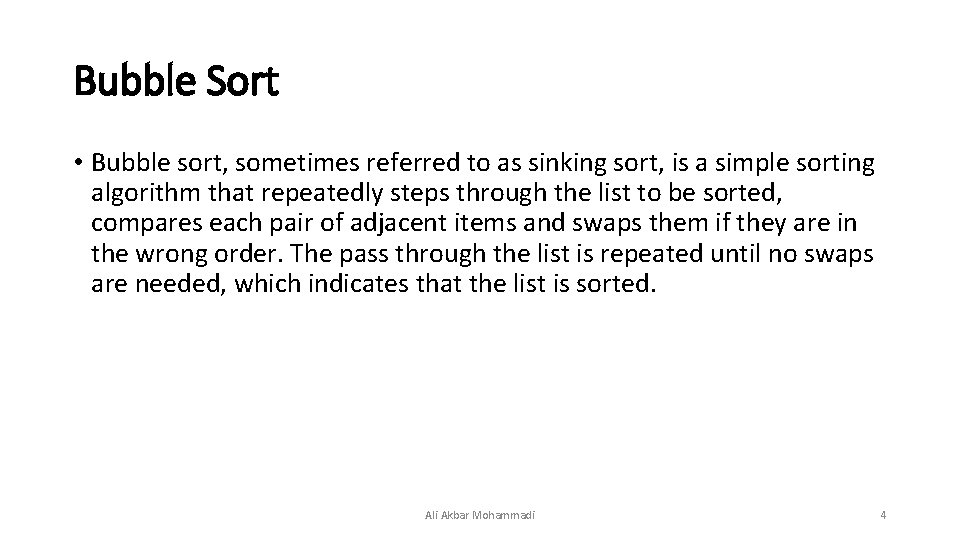Bubble Sort • Bubble sort, sometimes referred to as sinking sort, is a simple sorting algorithm that repeatedly steps through the list to be sorted, compares each pair of adjacent items and swaps them if they are in the wrong order. The pass through the list is repeated until no swaps are needed, which indicates that the list is sorted. Ali Akbar Mohammadi 4Bubble Sort Ali Akbar Mohammadi 5Performance Worst Case Best Case Average Case О(n 2) Ali Akbar Mohammadi 6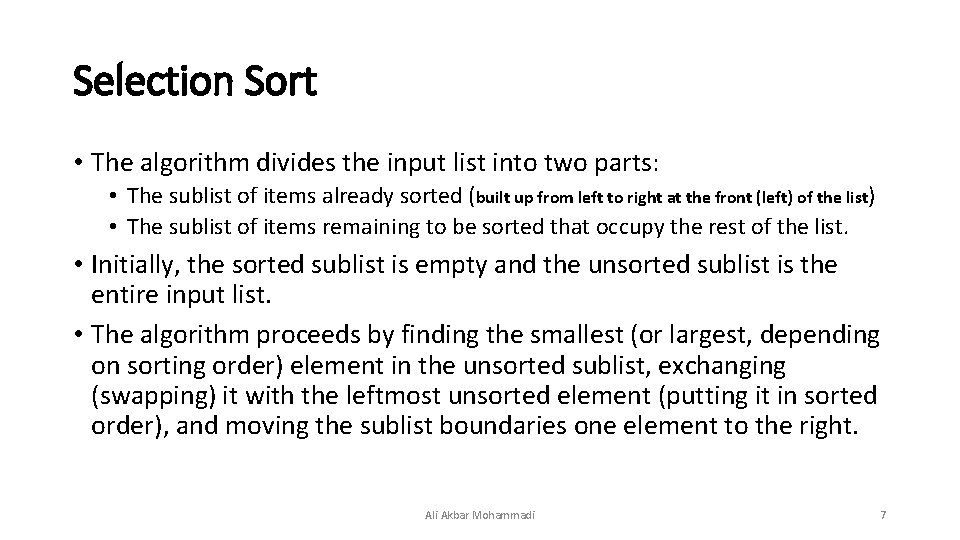Selection Sort • The algorithm divides the input list into two parts: • The sublist of items already sorted (built up from left to right at the front (left) of the list) • The sublist of items remaining to be sorted that occupy the rest of the list. • Initially, the sorted sublist is empty and the unsorted sublist is the entire input list. • The algorithm proceeds by finding the smallest (or largest, depending on sorting order) element in the unsorted sublist, exchanging (swapping) it with the leftmost unsorted element (putting it in sorted order), and moving the sublist boundaries one element to the right. Ali Akbar Mohammadi 7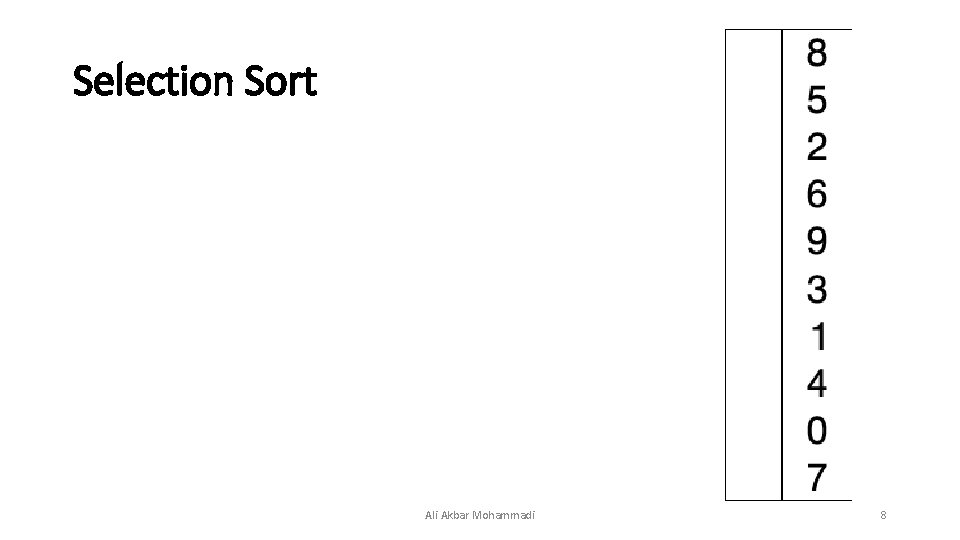Selection Sort Ali Akbar Mohammadi 8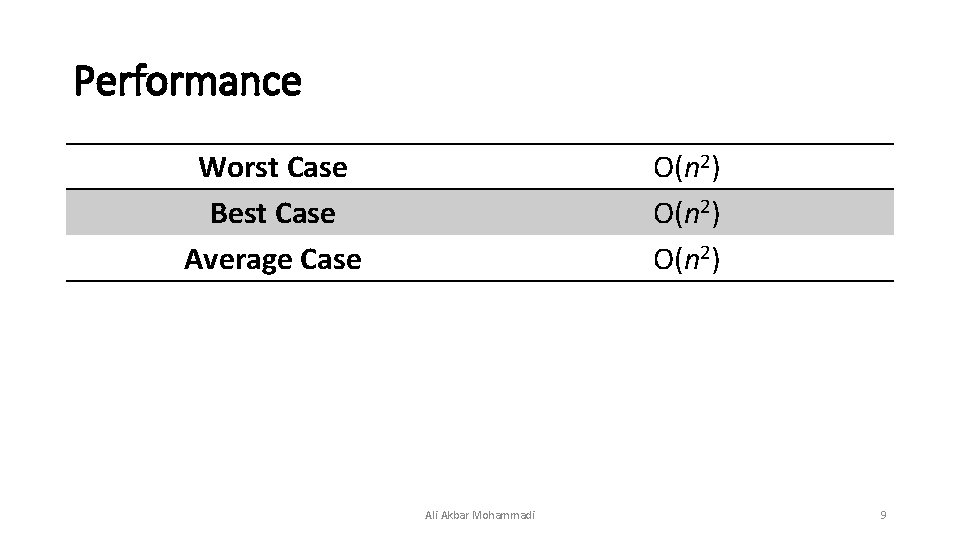Performance Worst Case Best Case Average Case О(n 2) Ali Akbar Mohammadi 9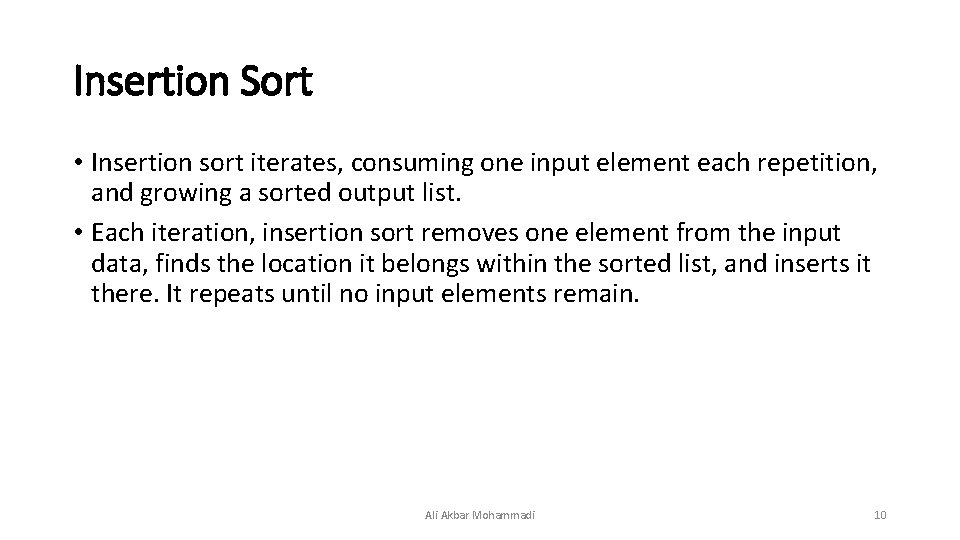Insertion Sort • Insertion sort iterates, consuming one input element each repetition, and growing a sorted output list. • Each iteration, insertion sort removes one element from the input data, finds the location it belongs within the sorted list, and inserts it there. It repeats until no input elements remain. Ali Akbar Mohammadi 10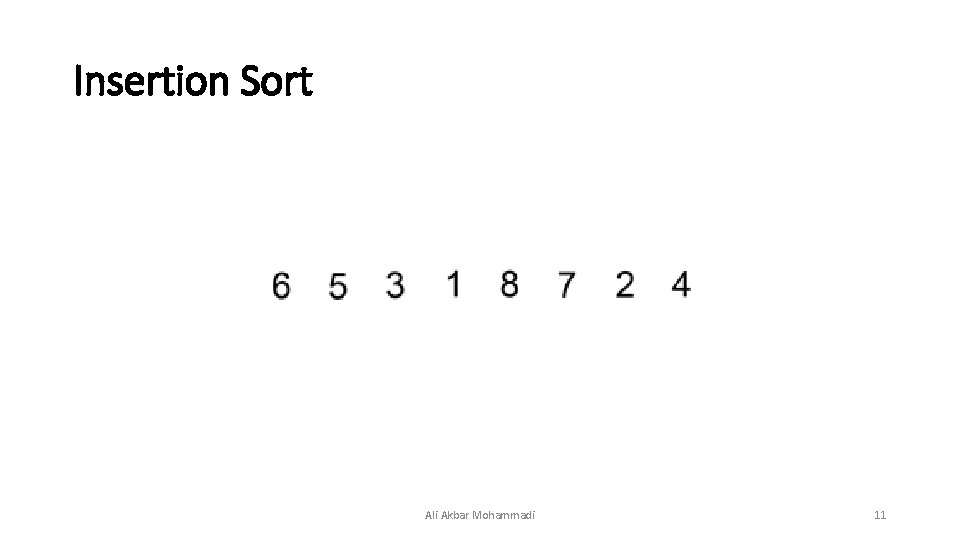Insertion Sort Ali Akbar Mohammadi 11Performance Worst Case Best Case Average Case О(n 2) Ali Akbar Mohammadi 12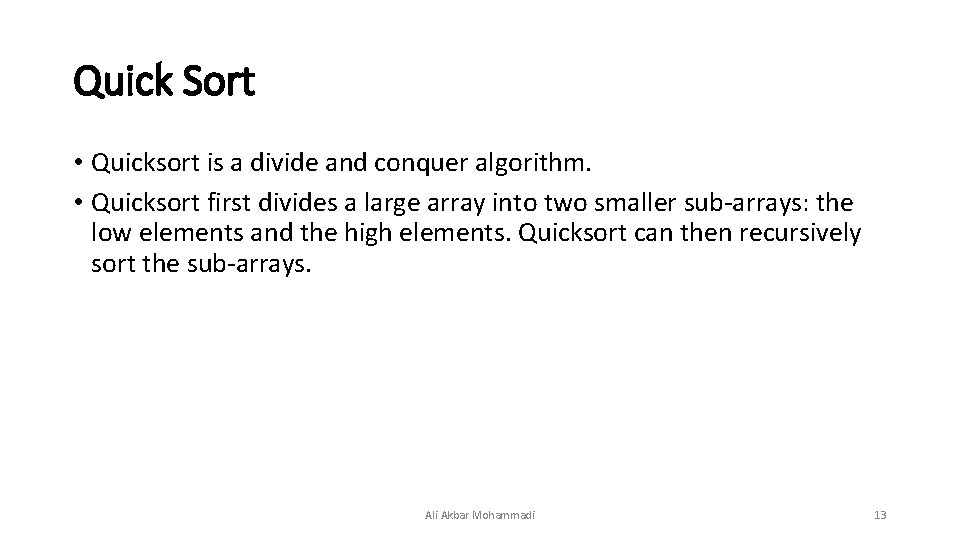Quick Sort • Quicksort is a divide and conquer algorithm. • Quicksort first divides a large array into two smaller sub-arrays: the low elements and the high elements. Quicksort can then recursively sort the sub-arrays. Ali Akbar Mohammadi 13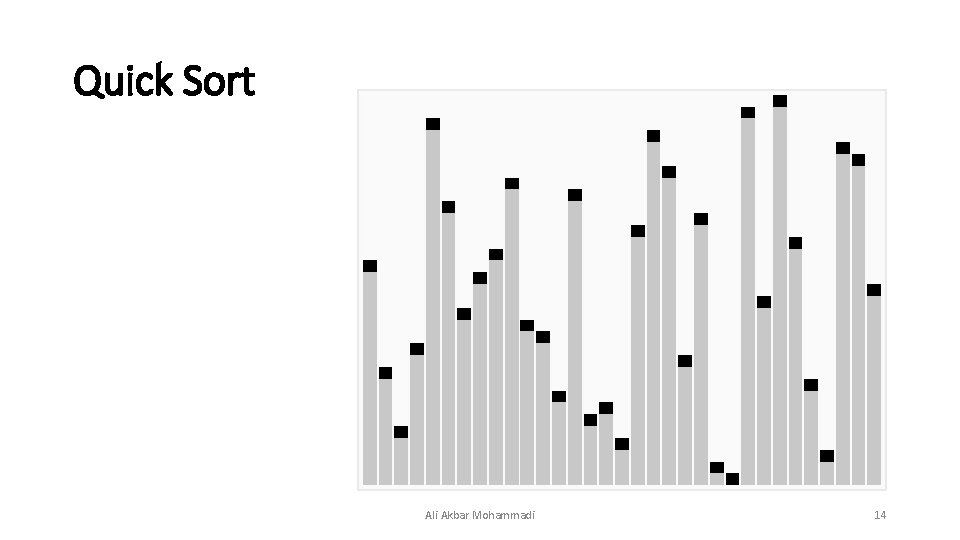Quick Sort Ali Akbar Mohammadi 14Performance Worst Case Best Case Average Case O(n 2) O(n log n) Ali Akbar Mohammadi 15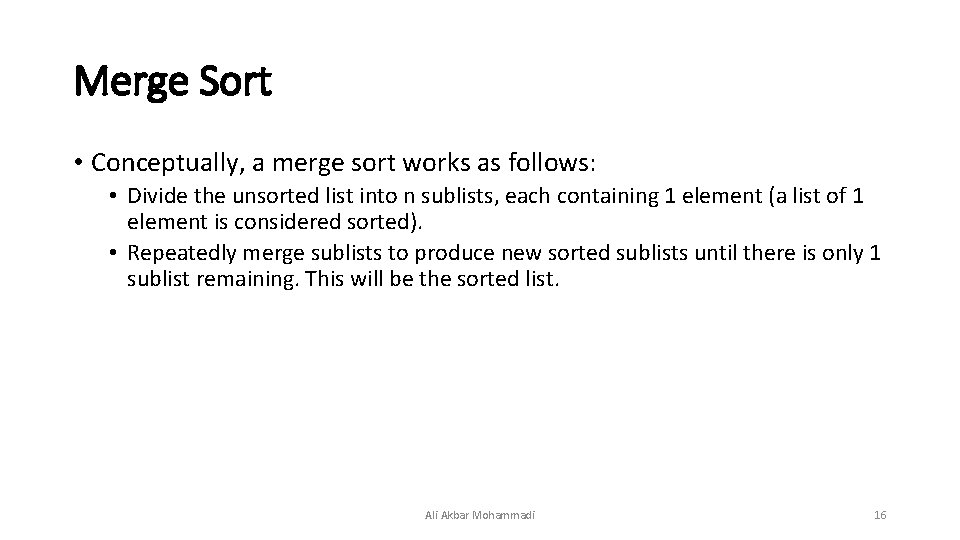Merge Sort • Conceptually, a merge sort works as follows: • Divide the unsorted list into n sublists, each containing 1 element (a list of 1 element is considered sorted). • Repeatedly merge sublists to produce new sorted sublists until there is only 1 sublist remaining. This will be the sorted list. Ali Akbar Mohammadi 16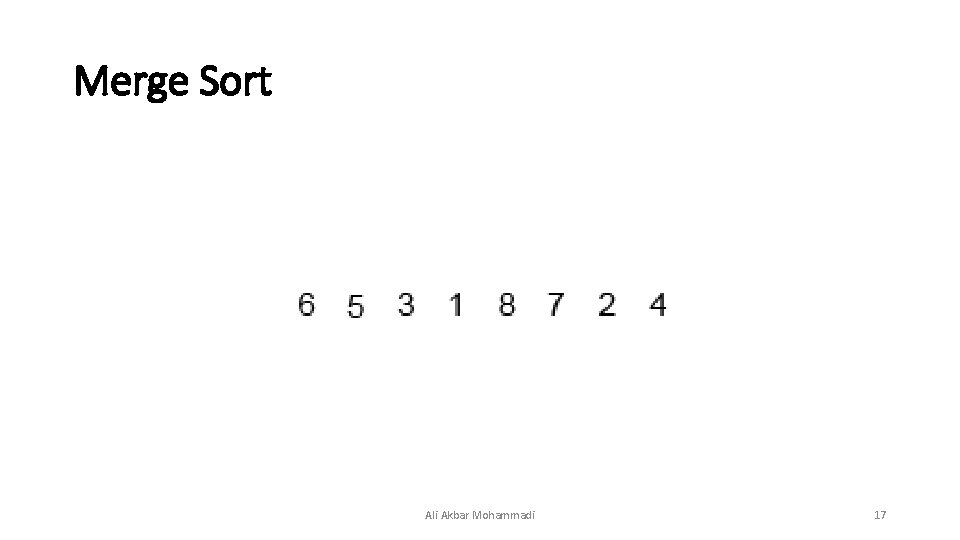Merge Sort Ali Akbar Mohammadi 17Performance Worst Case Best Case Average Case O(n log n) Ali Akbar Mohammadi 18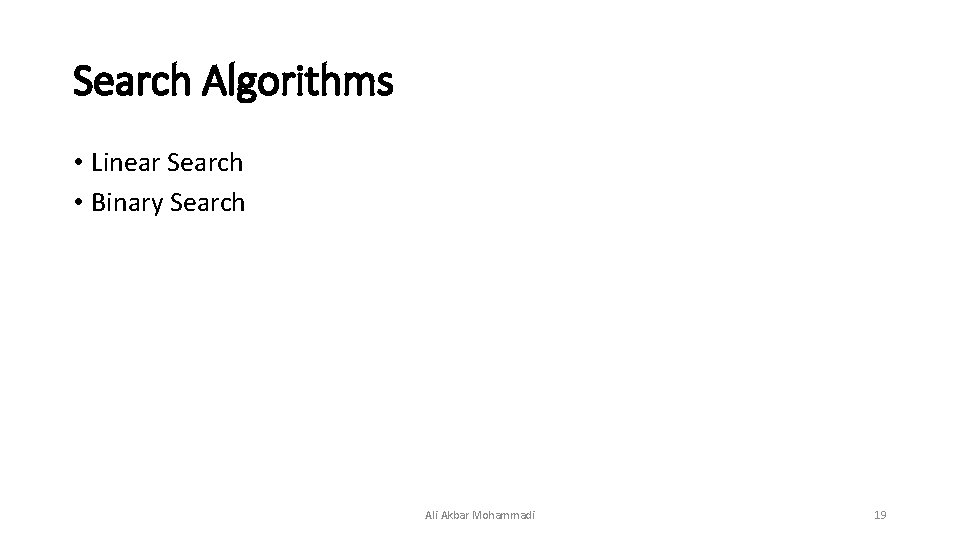Search Algorithms • Linear Search • Binary Search Ali Akbar Mohammadi 19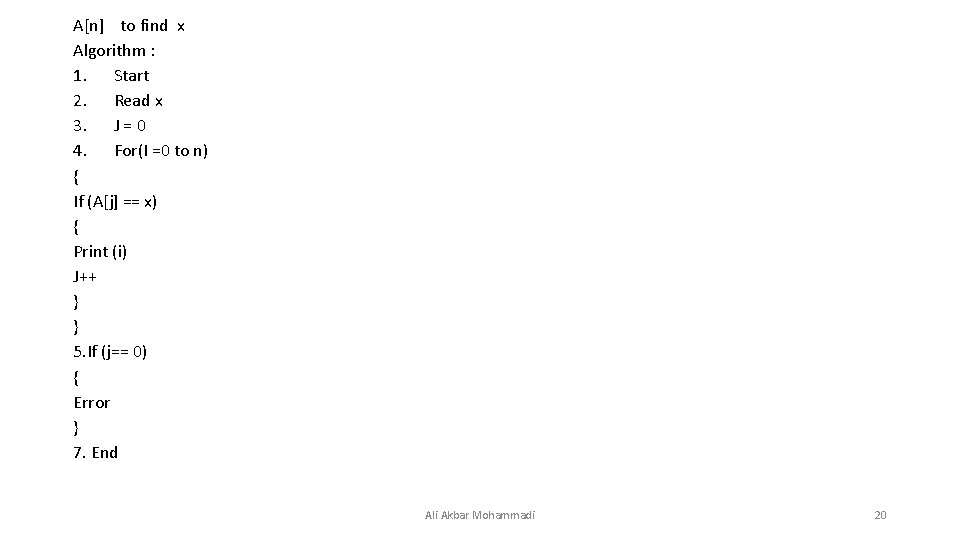A[n] to find x Algorithm : 1. Start 2. Read x 3. J = 0 4. For(I =0 to n) { If (A[j] == x) { Print (i) J++ } } 5. If (j== 0) { Error } 7. End Ali Akbar Mohammadi 20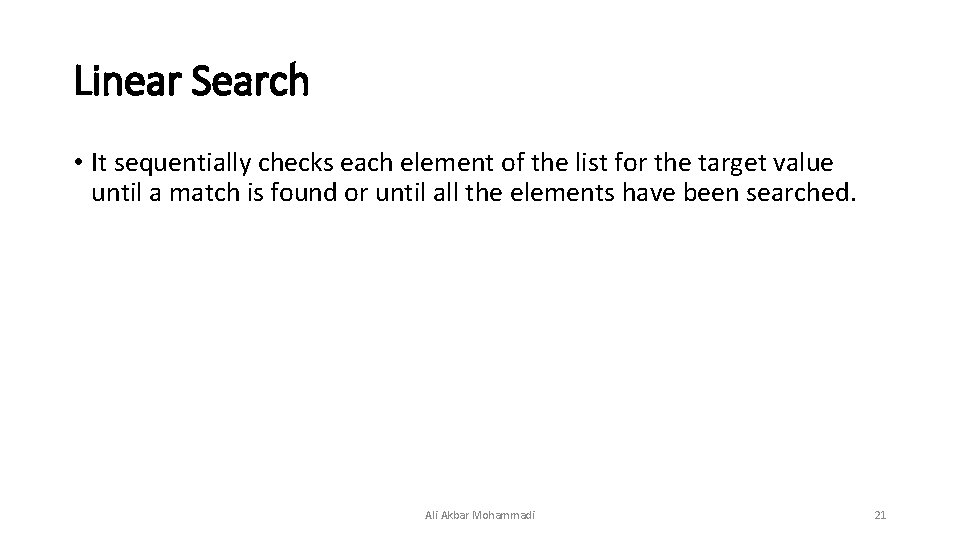Linear Search • It sequentially checks each element of the list for the target value until a match is found or until all the elements have been searched. Ali Akbar Mohammadi 21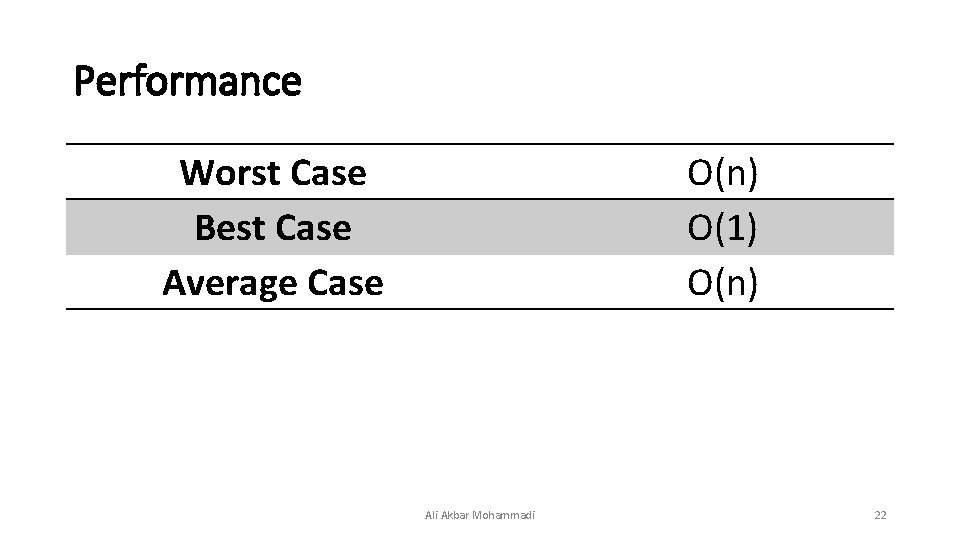Performance Worst Case Best Case Average Case O(n) O(1) O(n) Ali Akbar Mohammadi 22Binary Search • Binary search works on sorted arrays. • A binary search begins by comparing the middle element of the array with the target value. • If the target value matches the middle element, its position in the array is returned. • If the target value is less than or greater than the middle element, the search continues in the lower or upper half of the array, respectively, eliminating the other half from consideration. Ali Akbar Mohammadi 23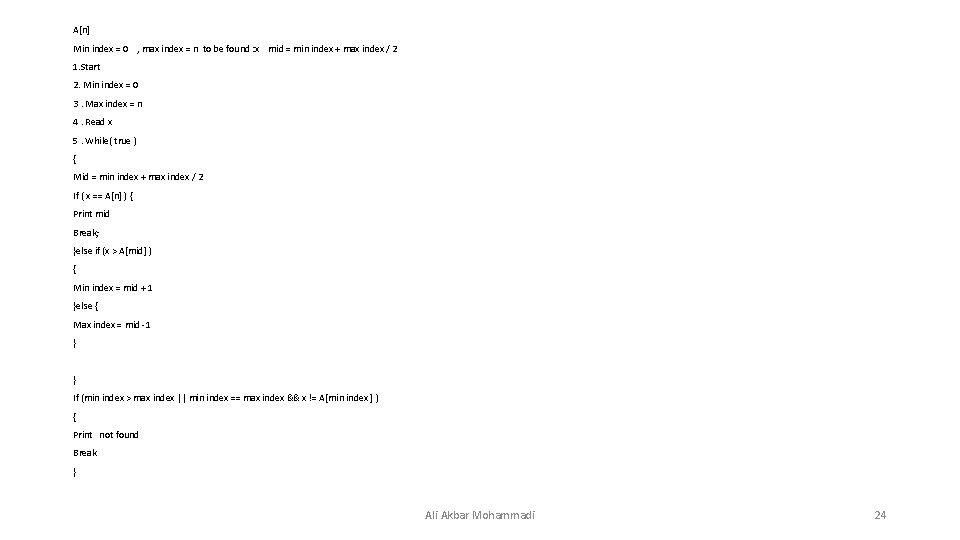A[n] Min index = 0 , max index = n to be found : x mid = min index + max index / 2 1. Start 2. Min index = 0 3. Max index = n 4. Read x 5. While( true ) { Mid = min index + max index / 2 If ( x == A[n] ) { Print mid Break; }else if (x > A[mid] ) { Min index = mid + 1 }else { Max index = mid -1 } } If (min index > max index || min index == max index && x != A[min index ] ) { Print not found Break } Ali Akbar Mohammadi 24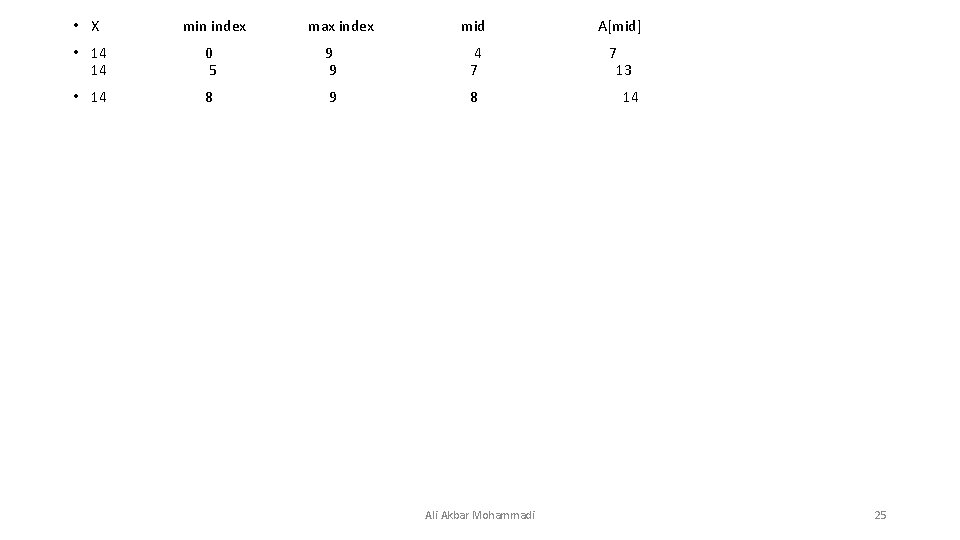• X min index max index mid A[mid] • 14 0 9 4 7 14 5 9 7 13 • 14 8 9 8 14 Ali Akbar Mohammadi 25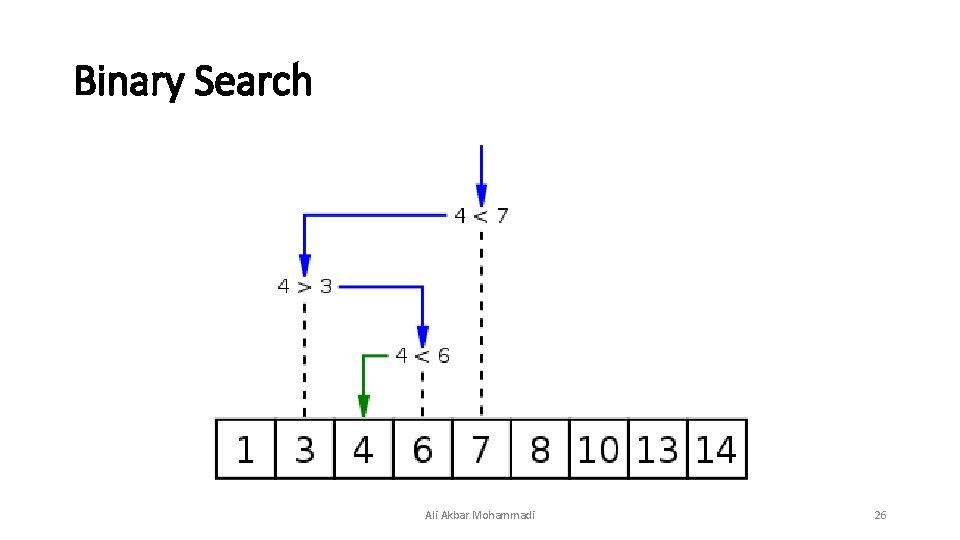Binary Search Ali Akbar Mohammadi 26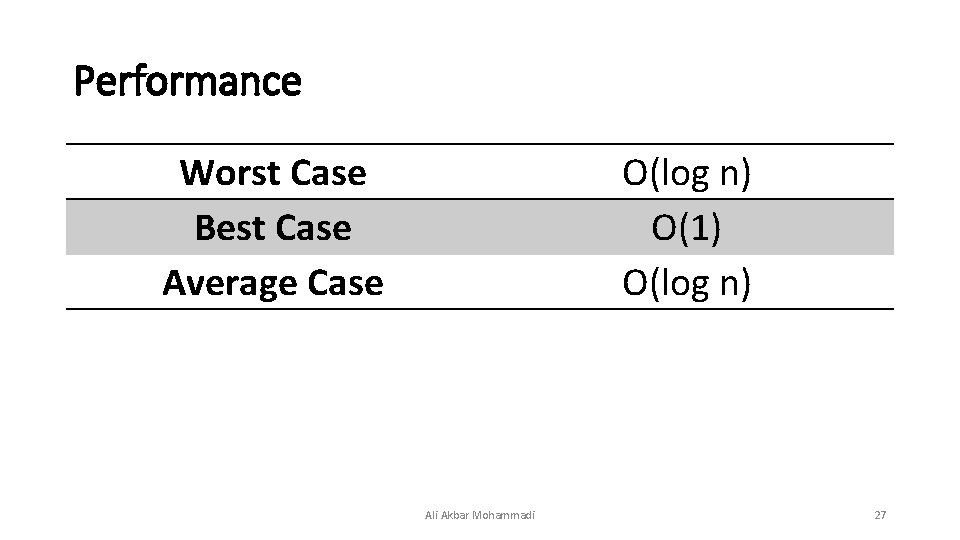Performance Worst Case Best Case Average Case O(log n) O(1) O(log n) Ali Akbar Mohammadi 27